# 零知识 QAP问题的转化

## 术语介绍

P 问题 指的是在多项式时间内可解的问题。

NP 问题 （Non-Deterministic Polynomial Problem，非确定性多项式问题），指不能在多项式内可解，但是可以在多项式时间内验证的问题。

QSP 问题 （QSP – Quadratic Span Program），实现基于布尔电路的 NP 问题的证明和验证。

QAP 问题 (Quadratic Arithmetic Program)，实现基于算术电路的 NP 问题的证明和验证，相对于 QSP，QAP 有更好的普适性。

## 正文翻译

1- 你知道zk-SNARKs和他的大致原理；（译者注：如果不知道zk-SNARK，建议您可以参考《一个数独引发的惨案：零知识证明》）

2- 你有足够的数学知识，能理解一些基本的多项式知识。(如 `if P(x)+ Q(x)=(P + Q)(x)``P``Q`代表多项式，如果你对这类多项式表述方式已经非常熟悉，说明你符合继续阅读的要求)。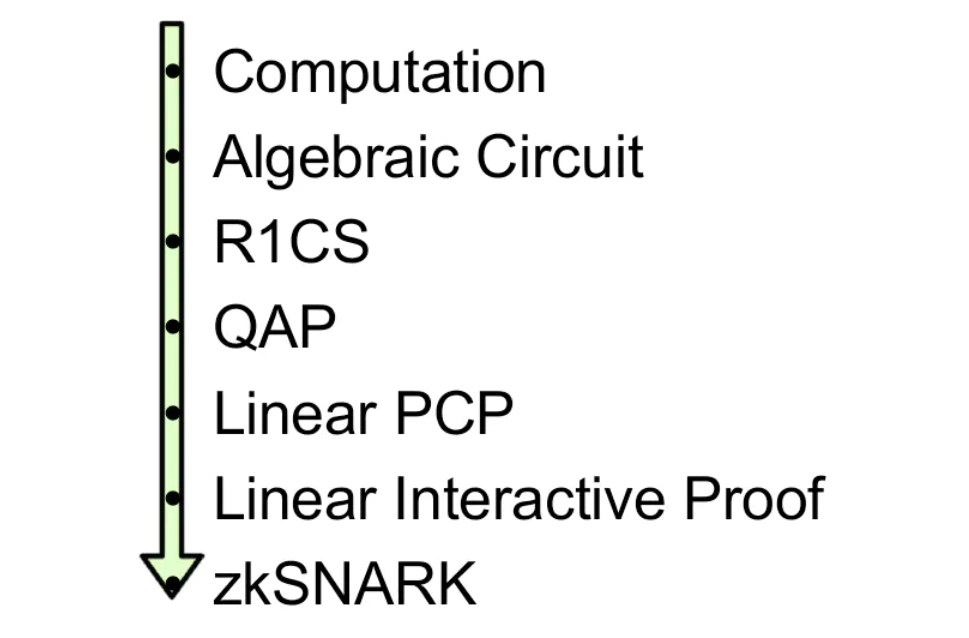``````def qeval(x):

y = x**3

return x + y + 5``````

### 第一步：压扁

1. `x = y` （y可以是变量或数字)
2. `x = y(op)z` (op可以+,-,*,/，y和z可以是变量，数字或子表达式)。

``````sym_1 = x * x

y = sym_1 * x //相当于实现了幂函数y = x**3

sym_2 = y + x

~out = sym_2 + 5``````

### 第二步：转为R1CS

``s . a * s . b - s . c = 0``

``````a = (5,0,0,0,0,1)，

b = (1,0,0,0,0,0)，

c = (0,0,1,0,0,0)，

s = (1,3,35,9,27,30)，``````（译者注：第一个`35=1*5 + 30*1`，第二个`35=35 * 1`

``'~one', 'x', '~out', 'sym_1', 'y', 'sym_2'``

#### 第一个门

``sym_1 = x * x，即 x*x - sym_1 = 0``

``````a = [0, 1, 0, 0, 0, 0]

b = [0, 1, 0, 0, 0, 0]

c = [0, 0, 0, 1, 0, 0]``````

#### 第二个门

``y = sym_1 * x，即 sym_1 * x - y = 0``

``````a = [0, 0, 0, 1, 0, 0]

b = [0, 1, 0, 0, 0, 0]

c = [0, 0, 0, 0, 1, 0]``````

#### 第三个门

``sym_2 = y + x，加法门需要转换为：(x + y) * 1 - sym_2 = 0``

``````a = [0, 1, 0, 0, 1, 0] 对应(x + y)

b = [1, 0, 0, 0, 0, 0] 对应常量1，用~one位

c = [0, 0, 0, 0, 0, 1]``````

#### 第四个门

``~out = sym_2 + 5，即 (sym_2 + 5) * 1 - ~out = 0``

``````a = [5, 0, 0, 0, 0, 1]

b = [1, 0, 0, 0, 0, 0]

c = [0, 0, 1, 0, 0, 0]``````

``s = [1, 3, 35, 9, 27, 30]``

``````A

[0, 1, 0, 0, 0, 0]

[0, 0, 0, 1, 0, 0]

[0, 1, 0, 0, 1, 0]

[5, 0, 0, 0, 0, 1]

B

[0, 1, 0, 0, 0, 0]

[0, 1, 0, 0, 0, 0]

[1, 0, 0, 0, 0, 0]

[1, 0, 0, 0, 0, 0]

C

[0, 0, 0, 1, 0, 0]

[0, 0, 0, 0, 1, 0]

[0, 0, 0, 0, 0, 1]

[0, 0, 1, 0, 0, 0]``````

### 第三步：从R1CS 到 QAP

``y = (x - 2) * (x - 3)``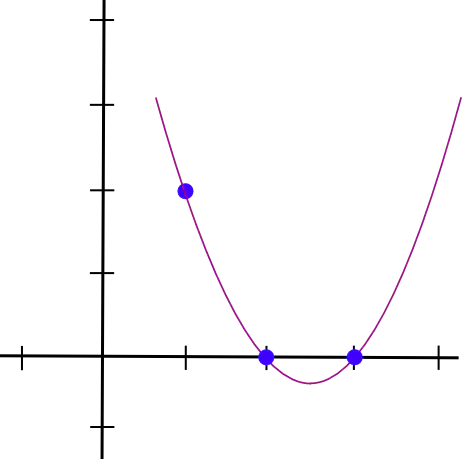``y = (x - 2) * (x - 3) * 3 / ((1 - 2) * (1 - 3))``

``y = 1.5 * x**2 - 7.5 * x + 9``

``y = 1.5 * x**2 - 5.5 * x + 7``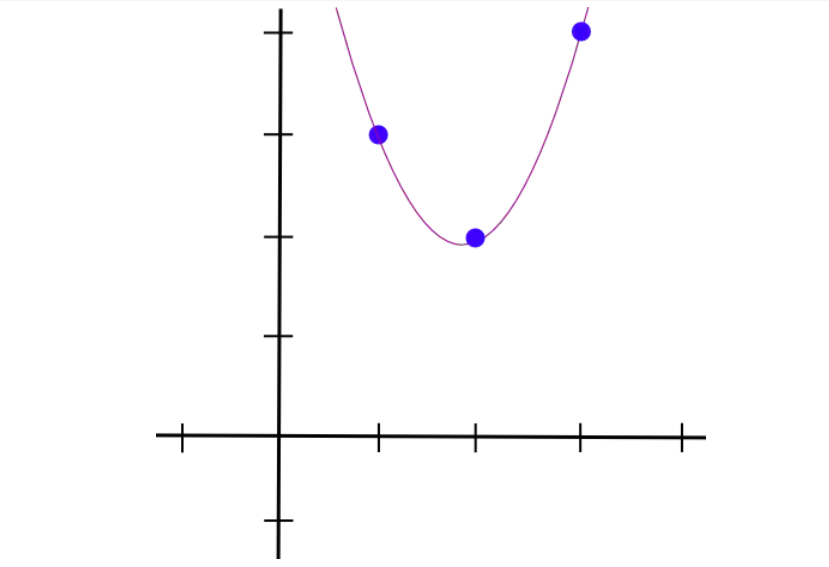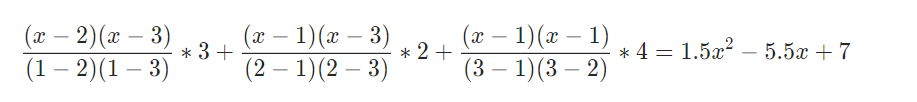``````A polynomials

[-5.0, 9.166, -5.0, 0.833]

[8.0, -11.333, 5.0, -0.666]

[0.0, 0.0, 0.0, 0.0]

[-6.0, 9.5, -4.0, 0.5]

[4.0, -7.0, 3.5, -0.5]

[-1.0, 1.833, -1.0, 0.166]

B polynomials

[3.0, -5.166, 2.5, -0.333]

[-2.0, 5.166, -2.5, 0.333]

[0.0, 0.0, 0.0, 0.0]

[0.0, 0.0, 0.0, 0.0]

[0.0, 0.0, 0.0, 0.0]

[0.0, 0.0, 0.0, 0.0]

C polynomials

[0.0, 0.0, 0.0, 0.0]

[0.0, 0.0, 0.0, 0.0]

[-1.0, 1.833, -1.0, 0.166]

[4.0, -4.333, 1.5, -0.166]

[-6.0, 9.5, -4.0, 0.5]

[4.0, -7.0, 3.5, -0.5]``````

（译者注：以上计算过程： 将 (1,0), (2,0), (3,0), (4,5)，带入拉格朗日插值公式计算得出，第二个多项式则是将 (1,1),(2,0),(3,1),(4,0) 带入拉格朗日插值公式计算得出, 由此类推）

``````A results at x=1
0
1
0
0
0
0
B results at x=1
0
1
0
0
0
0
C results at x=1
0
0
0
1
0
0``````

（译者注：以上计算过程： x=1 代入第一个多项式`0.833 * x**3 - 5 * x**2 + 9.166 * x - 5` = 0，x=1代入第二个多项式`-0.666 * x**3 + 5 * x**2 - 11.333 * x + 8` =1, 由此类推）

### 第四步：检查QAP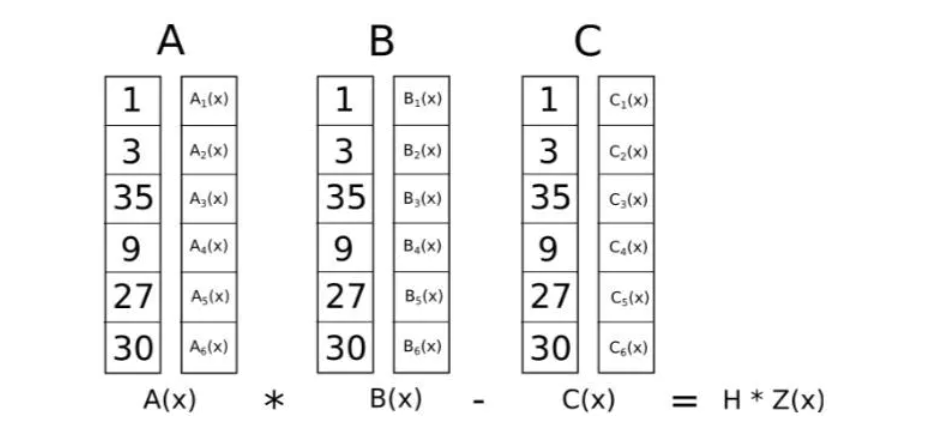Z定义为(x – 1) (x – 2) (x – 3)…-最简单的多项式，在所有对应逻辑门的点上都等于0。这是代数的一个基本事实任何多项式在所有这些点上等于零都必须是这个最小多项式的倍数，如果一个多项式是Z的倍数那么它在任何这些点上的值都是零；这种对等使我们的工作容易得多。

``````A . s = [43.0, -73.333, 38.5, -5.166]
B . s = [-3.0, 10.333, -5.0, 0.666]
C . s = [-41.0, 71.666, -24.5, 2.833]``````

（译者注：以上计算过程：

43.0 = -5 1 + 8 3 + 0 35 – 6 9 + 4 27 – 1 30，

-73.333 = 9.166 1 – 11.333 3 + 0 35 + 9.5 9 – 7 27 + 1.833 30,

-3 = 3 1 – 2 3 + 0 35 + 0 9 + 0 27 + 0 30

…)

t = [-88.0, 592.666, -1063.777, 805.833, -294.777, 51.5, -3.444]

（译者注：计算过程：

A . s = [43.0, -73.333, 38.5, -5.166] = -5.166 * x*3 + 38.5 x*2 – 73.333 x + 43,

B . s = [-3.0, 10.333, -5.0, 0.666] = 0.666 * x*3 – 5 x*2 + 10.333 x – 3.0,

C . s = [-41.0, 71.666, -24.5, 2.833] = 2.833 * x*3 – 24.5 x*2 + 71.666 x – 41.0

A . s * B . s – C . s 就是上面多项式的计算，计算后，按幂从低到高排列系数，得到： [-88.0, 592.666, -1063.777, 805.833, -294.777, 51.5, -3.444]

``Z = (x - 1) * (x - 2) * (x - 3) * (x - 4)``

``Z = [24, -50, 35, -10, 1]``

``h = t / Z = [-3.666, 17.055, -3.444]``

h没有任何余数

• 发表于 2022-05-30 16:32
• 阅读 ( 514 )
• 学分 ( 11 )
• 分类：零知识证明